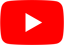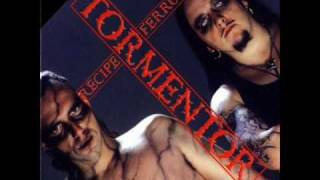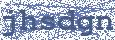Dracula
Tormentor

## TORMENTOR

Dracula Lyrics

We, we are the truth
We, we are the light
We, we are the love
We, we are the god

We, we are the bats
We, we are the wolfs
We, we are the bears
We, we are the boars

Now we will forge the power
Now our sun will rise again
Our mind in the sky
Our eye in the sun

We, we are the earth
We, we are the water
We, we are the air
We, we are the fire

We, we are the stones
We, we are the plants
We, we are the animals
We, we are the humans

We, we are the finest
We, we are the deepest
We, we are the greatest
We, we are the darkest

We, we are the moon
We, we are the space
We, we are the hellHottest Lyrics with Videos
6628529f5c17c646dc29f4b3ea81da13